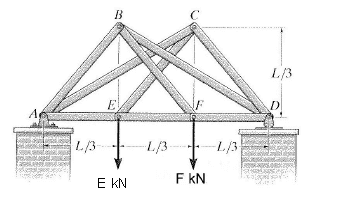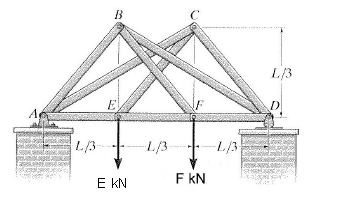# Complex scissors truss question

## Homework StatementThe symmetrical scissors truss is supported at point A and D. The support at D is a rocker bearing and does not produce a horizontal reaction. A, B, C, D, E, & F are pin joints. The members of the truss are not joined to each other where they cross. At A, the angle between AB and AE is 45 degrees and the angle between AC and AE is 26.57 degrees. The magnitude of the two loads, E and F are given as 192.6 kN and 135.8 kN respectively. L is equal to 3m.

What is the magnitude of the reaction force at D?

If the load at F has changed so that the vertical reaction at A is 125.4 kN:

what is the magnitude of the axial force in members AB, CD and AE?

## Homework Equations

Sum of all moments = 0
Sum of all forces = 0
Sum Fx = 0
Sum Fy = 0
M=Fd

## The Attempt at a Solution

I worked out the three moment equations about B, C and E, however I have got too many variables to solve the equations.

PhanthomJay
Homework Helper
Gold Member

## Homework StatementThe symmetrical scissors truss is supported at point A and D. The support at D is a rocker bearing and does not produce a horizontal reaction. A, B, C, D, E, & F are pin joints. The members of the truss are not joined to each other where they cross. At A, the angle between AB and AE is 45 degrees and the angle between AC and AE is 26.57 degrees. The magnitude of the two loads, E and F are given as 192.6 kN and 135.8 kN respectively. L is equal to 3m.

What is the magnitude of the reaction force at D?

If the load at F has changed so that the vertical reaction at A is 125.4 kN:

what is the magnitude of the axial force in members AB, CD and AE?

## Homework Equations

Sum of all moments = 0
Sum of all forces = 0
Sum Fx = 0
Sum Fy = 0
M=Fd

## The Attempt at a Solution

I worked out the three moment equations about B, C and E, however I have got too many variables to solve the equations.
The first part of the question asks you to determine the reaction at D. Have you done this part yet by summing moments about A? For part 2, under a different loading case, you are asked to find member forces. It's usually best, after determining support reactions, to look at the easier joints first, which in this case are E and F. You can solve then for the diagonal member forces emanating from those joints, and the rest of the forces can then be calculated.

Would the sum of the moments about A just be:
(192.6 x 1) + (135.8 x 2)
?
And so the reaction at D is the sum of the moments at A/3?

PhanthomJay
Homework Helper
Gold Member
Would the sum of the moments about A just be:
(192.6 x 1) + (135.8 x 2)
?
And so the reaction at D is the sum of the moments at A/3?
I don't know what you mean by A/3. You know that the sum of the moments about any point must be zero. If you choose joint A as the point about which you sum moments, then the reaction at A will not enter into the equation, because it produces no moment about point A. So representing the vertical reaction at D as an upward force $$D_y$$, your equation becomes
$$\Sigma M_A = 0$$
$$192.6(L/3) + 135.8(2L/3) - D_y(?) = 0$$ (you fill in what to use for (?)).
Edit: I re-read your post and it looks like we are saying the same thing, D_y = (sum of moments about A from the applied loads at E and F)/3

Last edited:
ah cool, thank you very much, that explains lots

what I meant by A/3 was that:
because sum of moments at A = 0, and L =3

Dy x 3 = (192.6 x 1) + (135.8 x 2)
therefore:

Dy = [(192.6 x 1) + (135.8 x 2)] /3

With the vertical reaction at A has different for Part 2, do I sum the y-components to find the load at F?

0 = Dy + Ay - E - F ?

Last edited:
PhanthomJay
Homework Helper
Gold Member
ah cool, thank you very much, that explains lots

what I meant by A/3 was that:
because sum of moments at A = 0, and L =3

Dy x 3 = (192.6 x 1) + (135.8 x 2)
therefore:

Dy = [(192.6 x 1) + (135.8 x 2)] /3
yes this is OK, you've assumed L=3, but actually, L can be any number, and since it cancels out of the equation, it does not affect the analysis in this problem.
With the vertical reaction at A has different for Part 2, do I sum the y-components to find the load at F?

0 = Dy + Ay - E - F ?
This equation is correct, but since both F and Dy are unknown, you need another equation before you can solve for F (Dy will not be the same as before). This 2nd equation comes from summing moments about D. Then you can get F right away, and then find Dy from your first equation.

ok thanks ^_^

Hey i'm new to this forum and am doin the exact same queastion but with different values, how would you find the members, using method of sections, i know you cannot cut through more than 3 members or cut through more than 2 braching from the same joint. How would I approach the rest of the question

hey guys this helped heaps..

LAgreek - heres how to find member CD

CD = E / sqrt(4.5)